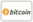Copertina di Numerical Methods in Meteorology
Titolo del libro:

# Numerical Methods in Meteorology

## Solved Problems

LAP LAMBERT Academic Publishing (15.02.2014 )eleggibile a buono
ISBN-13:

### 978-3-8443-9003-2

ISBN-10:
3844390030
EAN:
9783844390032
Lingua del libro:
Inglese
Risvolto di copertina:
This textbook presents an extensive collection of solved problems focusing on numerical methods used for the modeling of the atmosphere, following a traditional, Eulerian, grid-point, finite-differencing approach. It covers exercises related to the discrete representation of continuous functions, stability analysis of numerical solutions, time-differencing and linear advection. The most extensive part of the textbook is devoted to horizontal finite-differencing and the behavior of simulated gravity-inertial and Rossby waves on different horizontal distributions of variables. The simulation of nonlinear advection processes is also extensively covered. The textbook concludes with problems related to computationally economical schemes and generalized finite-differencing operators. MATLAB and FORTRAN are used to illustrate how to calculate numerical solutions and visualize the results. Students of meteorology, and experts engaged in the modeling of atmosphere and oceans can benefit from this textbook. In addition, professionals working in other science disciplines that require numerical solving of partial differential equations will find this book to be of interest.
Casa editrice:
Sito Web:
https://www.lap-publishing.com/
Da (autore):
Milivoj B. Gavrilov, Ivana A. Tošić, Miodrag Rančić
Numero di pagine:
172
Pubblicato il:
15.02.2014
Giacenza di magazzino:
Disponibile
categoria:
Geoscienze
Prezzo:
51,90 €
Parole chiave:
Atmospheric modeling, Arakawa grids, Arakawa Jacobian, Eulerian method, Gravity-inertia waves, Horizontal differencing, Numerical operators, Rossby waves, Time schemes### Categorie